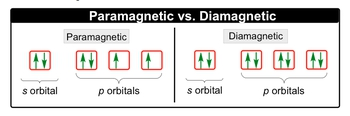## General Chemistry

Learn the toughest concepts covered in Chemistry with step-by-step video tutorials and practice problems by world-class tutors

10. Periodic Properties of the Elements

# Paramagnetism and Diamagnetism

Paramagnetism and Diamagnetism deal with the presence of electrons that are either unpaired or paired in an orbital.

Paramagnetism & Diamagnetism
1
concept

## Paramagnetism and Diamagnetism1m
Play a video:
now recall that in orbital can hold a maximum of two electrons that pair up with opposite spins. Remember, the opposite spins is part of the poly exclusion principle. Now we have two terms para magnetic versus dia Magnetic with para magnetic. This is one at least one electron and given orbital's is unp aired and die Magnetic is when all electrons and given orbital's are paired We're gonna realize that para magnetic substances are also magnetic meaning that they're influenced by a magnetic field. So if we take a look here para magnetic if we look we have an s orbital All the electrons within that one orbital are paired up. But if we look at the p orbital's we have this electron by itself in an orbital. So it's uncared and this one too as long as you have one electron in an orbital by itself um pared its para magnetic for dia magnetic If we look, all the electrons in the s orbital are paired up and then all the electrons in the P orbital's are paired up. There is not one electron by itself within a given orbital. So this is what is meant by Dia magnetic. So just keep this in mind when doing the electron configurations of either elements or ions. Para magnetic elements and ions have at least one electron by itself in an orbital somewhere. Dia magnetic. Every single orbital has two electrons in it. All of the electrons are therefore paired up.

A paramagnetic element has at least one unpaired electron and a diamagnetic element has no unpaired electrons.2
example

## Paramagnetism and Diamagnetism Example 11m
Play a video:
here, it says to determine if the vanadium Adam is para magnetic or diet magnetic, so Adam means that it's in its neutral form. Vanadium is represented by the If you look at the periodic table, its atomic number is 23. Here it's electron configuration would be are gone for us to three d one. If we look, we know that in S Orbital's there's only one orbital and they can hold a maximum of two electrons. So the four s orbital is already filled in with two electrons. R D sub level has five orbital's. And here there's only one electron there. So vanadium has one electron by itself within in orbital. So it's uncared, meaning that the vanadium Adam is para magnetic. So just remember, if you have a least one electron by itself in any given orbital, it's a para bathetic species.
3
Problem

Which of the following atoms has the most unpaired electrons?

4
Problem

Write the condensed electron configuration for the nickel (III) ion and state if it is paramagnetic or diamagnetic.